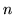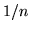Next: 16.4.4 Details Up: 16.4 Line Integral Convolution Previous: 16.4.2 Using OpenGL to   Contents

### 16.4.3 Line Integral Convolution Procedure

Next, make some simplifying assumptions to make the procedure simple:

1. The supplied flow field vector grid matches the tessellated textured surface; there's a one-to-one correspondence between vector and vertex.
2. Set a fixed number of displacements () at each vertex.

These assumptions allow you to simply use the vector associated with each vertex on the tessellated surface when computing texture displacements. You can also simply calculate the displacements by parameterizing the vector and computing evenly spaced texture coordinate locations displaced along the vector direction, both forwards and backwards.

Given these assumptions, the procedure looks like this:

1. Update the texture coordinates at each vertex on the surface.
2. Render the surface using the noise texture and the displaced texture coordinates.
3. Accumulate the resulting image in the accumulation buffer, scaling by.
4. Repeat the steps abovetimes, then return the accumulated image.
5. Perform histogram equalization or image scaling to maximize contrast.Next: 16.4.4 Details Up: 16.4 Line Integral Convolution Previous: 16.4.2 Using OpenGL to   Contents
2001-01-10